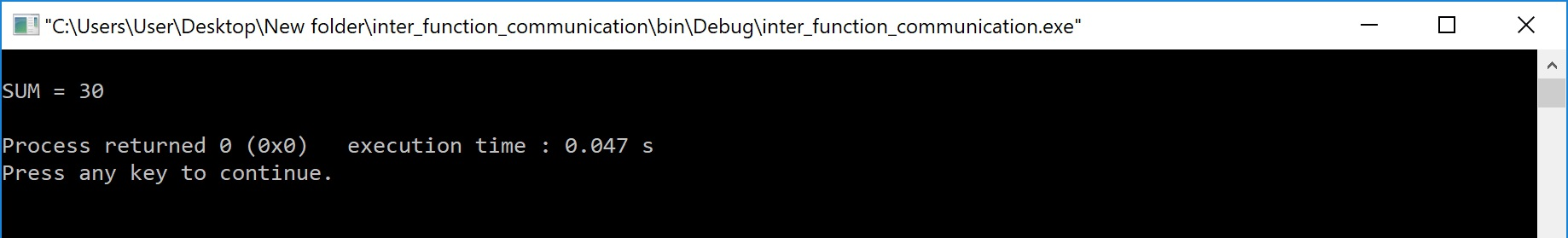The perfect place for easy learning...

×

# Inter Function Communication in C

When a function gets executed in the program, the execution control is transferred from calling a function to called function and executes function definition, and finally comes back to the calling function. In this process, both calling and called functions have to communicate with each other to exchange information. The process of exchanging information between calling and called functions is called inter-function communication.

In C, the inter function communication is classified as follows...

• Downward Communication
• Upward Communication
• Bi-directional Communication

# Downward Communication

In this type of inter function communication, the data is transferred from calling function to called function but not from called function to calling function. The functions with parameters and without return value are considered under downward communication. In the case of downward communication, the execution control jumps from calling function to called function along with parameters and executes the function definition,and finally comes back to the calling function without any return value. For example consider the following program...

# Example Program

``````#include<stdio.h>
#include<conio.h>

void main(){
int num1, num2 ;
void addition(int, int) ; // function declaration
clrscr() ;
num1 = 10 ;
num2 = 20 ;

printf("\nBefore swap: num1 = %d, num2 = %d", num1, num2) ;
addition(num1, num2) ; // calling function

getch() ;
}
void addition(int a, int b)  // called function
{

printf("SUM = %d", a+b) ;

}```
```

Output:# Upward Communication

In this type of inter-function communication, the data is transferred from called function to calling-function but not from calling-function to called-function. The functions without parameters and with return value are considered under upward communication. In the case of upward communication, the execution control jumps from calling-function to called-function without parameters and executes the function definition, and finally comes back to the calling function along with a return value. For example, consider the following program...

# Example Program

``````#include<stdio.h>
#include<conio.h>

void main(){
int result ;
int addition() ; // function declaration
clrscr() ;

result = addition() ; // calling function

printf("SUM = %d", result) ;
getch() ;
}
{
int num1, num2 ;
num1 = 10;
num2 = 20;
return (num1+num2) ;
}```
```

Output:# Bi - Directional Communication

In this type of inter-function communication, the data is transferred from calling-function to called function and also from called function to calling-function. The functions with parameters and with return value are considered under bi-directional communication. In the case of bi-directional communication, the execution control jumps from calling-function to called function along with parameters and executes the function definition and finally comes back to the calling function along with a return value. For example, consider the following program...

# Example Program

``````#include<stdio.h>
#include<conio.h>

void main(){
int num1, num2, result ;
int addition(int, int) ; // function declaration
clrscr() ;

num1 = 10 ;
num2 = 20 ;

result = addition(num1, num2) ; // calling function

printf("SUM = %d", result) ;
getch() ;
}
int addition(int a, int b)  // called function
{
return (a+b) ;
}```
```

Output: